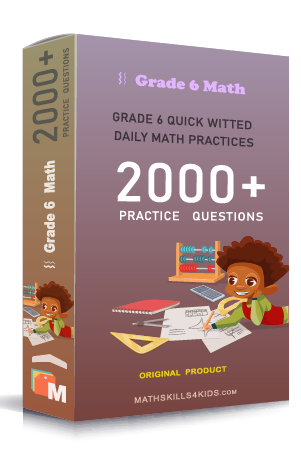# Grade 6 One Variable Equation worksheets with solutions

Subject
Math

Resource Type
Worksheets, Printables, Homeschool

Standards
CCSS 6.EE.B.5 | CCSS 6.EE.B.6 | CCSS 6.EE.B.7

Format
PDF (17.9 MB | 26 pages → Exercises + Solutions)

• ### -15% OFF Over \$100

Promo Code: TOPLA

After Purchase

\$3.00

This package allows you to practice the following skills

Does variable x satisfies an equation

Finding which variable x satisfies an equation

Writing an equation from words

Modeling and solving equations using algebra tiles

Writing and solving equations that represent diagrams

Solving one-step multiplication and division equations with whole numbers

Solving one-step addition and subtraction equations with whole numbers

Solving one-step equations with whole numbers

Solving one-step equations with decimals, fractions and mixed numbers

Solving one-step equations word problems

Solving one-step equations

Solving equations involving likes terms

Solving equations involving integers

This product is a part of the Mega Pack
* Grade 6 quick-witted daily math practices *